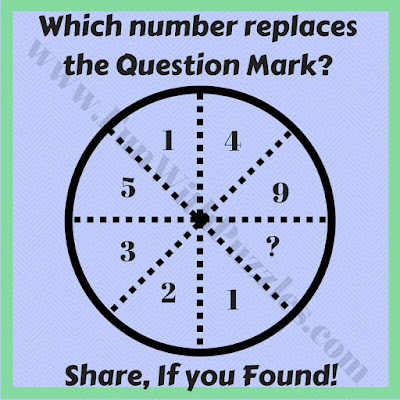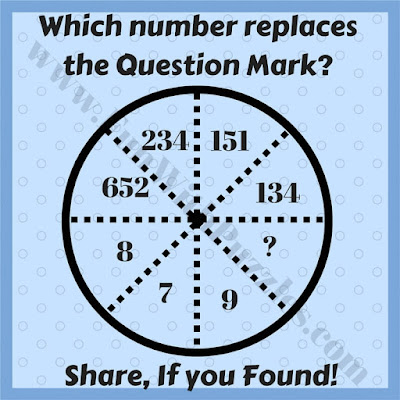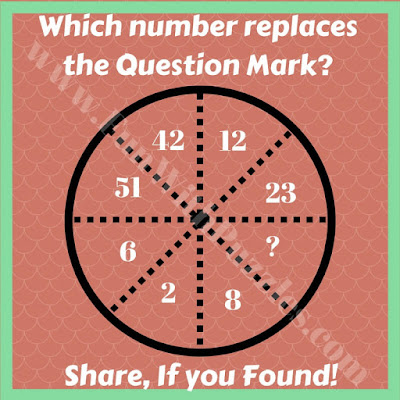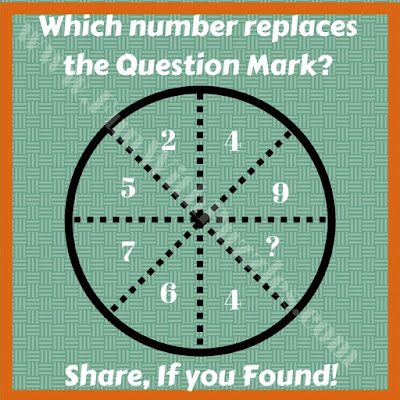## Monday, December 30, 2019

Mathematical and Logical Puzzles are very much fun to solve. In these kinds of puzzles, there will be some mathematical relationship between the numbers which follows a certain logical pattern. One has to find out this logical pattern and then solve it for the missing number. Circle Puzzles are one type of Mathematical and Logical Puzzles.
In these puzzles, the circle is divided into different segments. Each of the segment contains some numbers. There is some relationship between these numbers. One has to find these relations between the numbers in these circle segment and has to find the missing number in one of the segment.
These puzzles are arranged in the order of its difficulty, with the first one being the easiest one and the last one being the toughest one among the given set of puzzles. Few puzzles in the set are of the medium level of difficulty. Let us see how many of these puzzles you can solve?
Let us start with the easiest circle puzzle. This is the warm-up puzzle and even kids should be able to solve it very quickly.1. Easy circle math logic puzzle
After the easy Circle Puzzle, here is another easy quick easy puzzle question. Hope you will be able to solve this Circle puzzle very quickly :)2.Quick Circle math picture puzzle question
Next one is again not very tough and most of the people should be able to solve this Circle puzzle quickly.3. Fun circle logical reasoning puzzle question
After all, these easy and quick Circle puzzles here is the next puzzle question which may require some thinking. However again this is not a very tough puzzle. Let us see how quickly you can solve this puzzle?4. Circle math puzzle question
After all these easy puzzles, here come some challenges. However, if you are good in Mathematics and Logic then this puzzle also should not be very tough for you, but this puzzle could require some out of box thinking to solve it. You are a genius if you can solve this puzzle quickly.5. Tough circle logical puzzle

Do post your answers in the comment section of this post. There can always be different thinking which may lead to a different solution. Do post your answers with your logical thinking. It will be interesting to see if there is any different logic involved while solving these puzzles :)

1.1.